The purpose of the table below is to suggest general property ranges for comparison. This table does not reflect exact properties, nor should it be used for design purposes. It is the sole responsibility of the customer to determine whether a given tube will work for a given application. The strength and stiffness of composite tubing is very difficult to compare to metal tubing because of the possible variation in fiber orientation during layup. The strength, stiffness, and weight of composite tube is dictated by fiber modulus, fiber direction/placement, and resin system used. With metal tubing the properties are only dictated by the material type and dimension of the tubing itself. There is no directional element involved. This is actually one very large advantage composite tubing has over metal tubing. You will notice we have broken our chart down to show you the properties of the fibers by themselves and as an integrated epoxy resin/fiber matrix. This really shows how how critical the resin/fiber ratio is. Resin is necessary but too much resin can really weaken a tube structure. Our tubing has a resin/fiber ratio of about 33:67! You can't get much better than that. Below the chart you will find a glossary of terms used in our chart.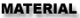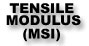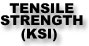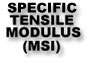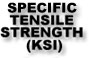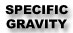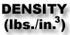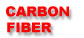33 580 18.3 322 1.8 .065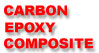17 275 11 178 1.54 .056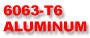10 36 3.7 13.3 2.71 .095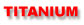15 170 3.25 36 4.62 .162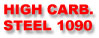30 122 3.8 15.6 7.82 .274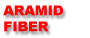18 400 12.5 278 1.44 .052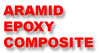9.8 190 7.5 146 1.30 .048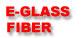10.5 500 4.1 197 2.54 .092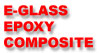6.5 257 3.25 129 1.99 .072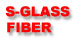12.5 665 5 267 2.49 .090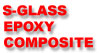7.7 342 4 175 1.95 .071

 GLOSSARY OF TERMS Tensile Modulus: Tensile modulus can be used as an indicator of the stiffness of a part. It is basically the applied tensile stress, based on the force and cross-sectional area, divided by the observed strain at that stress level. It is generally constant before the material approaches the point at which permanent deformation will begin to occur. It is most easily observed as the slope of the stress-strain curve prior to the yield point. In our chart the tensile modulus is shown as (MSI), or million pounds per square inch. Tensile modulus can also be shown as (10^6 PSI). Tensile Strength: The ultimate tensile strength is defined as the maximum stress that a material can withstand before failure in tension. Values are determined by an extension test. A simple example of tension would be the rope used in tension during a tug-of-war. Tensile strength tests are a common way to compare the strength of two materials. In the chart above tensile strength is displayed as (KSI), or thousand pounds per square inch. Carbon fiber is used most efficiently when loaded in tension. When a tube is loaded in bending some of the fibers experience tension while others experience compression. Specific Tensile Modulus: Specific tensile modulus can best be described as the stiffness to weight ratio of a given material. In our chart this number is determined by dividing the tensile modulus by its specific gravity (weight). It is an easy way to tell which material gives you more stiffness with the least weight penalty. Kevlar is the only material that even comes close to carbon fiber. Specific Tensile Strength: Specific tensile strength is the strength to weight ratio of a given material. In our chart this number is determined by dividing the tensile strength by its specific gravity (weight). Specific Gravity: Specific gravity is the heaviness of a substance compared to that of water, and it is expressed without units. If something is 7.82 times as heavy as an equal volume of water (such as 1090 steel is) its specific gravity is 7.82. Now you can really see how light carbon fiber is compared to other materials. Density: One of the fundamental properties of any material is its heaviness. In solids we think of materials like cork or styrofoam as being very light and lead and iron as being heavy. Actually we have to consider the volumes in this discussion. We could have a ton of styrofoam, or a half-gram of lead. It depends on how large a sample we are using. To calculate heaviness or density we divide the mass of material in pounds by its volume in cubic inches. Density could also be expressed as grams per cubic centimeter (g/cm3)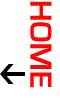Copyright 2023 Carbon Fiber Tube Shop